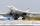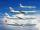Truck PRG-BA

Truck driving on highway from Prague to Bratislava at average speed of 72 km/h. At the moment when the truck passes 54 kilometer from Prague passenger car departs from Prague which travel to Bratislava at speed 90 km/h. When and where (at waht highway kilometer) passenger car catch truck?

Result

t =  3 h
s =  270 km

Solution:

s = 54 + 72 t
s = 90 t

s-72t = 54
s-90t = 0

s = 270
t = 3

Calculated by our linear equations calculator.

Leave us a comment of this math problem and its solution (i.e. if it is still somewhat unclear...):Be the first to comment!To solve this verbal math problem are needed these knowledge from mathematics:

Do you have a system of equations and looking for calculator system of linear equations?

Next similar math problems:

1. Blackjack TU160Bomber Tupolev TU160 started at 9:00 from the airport and flew at average speed of 1850 km/h toward the airfield B distant 3500 km. After evaluating the danger from the airport B started at 9:45 B towards A fighter MIG 29 at an average speed 2300 km/h. At
2. AircraftsAbove the town hall tower flew the plane with constant speed 592 km/h and 15 minutes later the second plane at speed of 675 km/h. How long and how far from the town hall will be aircrafts caught up?
3. Theorem proveWe want to prove the sentence: If the natural number n is divisible by six, then n is divisible by three. From what assumption we started?
4. Linear systemSolve a set of two equations of two unknowns: 1.5x+1.2y=0.6 0.8x-0.2y=2
5. LegsCancer has 5 pairs of legs. The insect has 6 legs. 60 animals have a total of 500 legs. How much more are cancers than insects?
6. Factory and divisionsThe factory consists of three auxiliary divisions total 2,406 employees. The second division has 76 employees less than 1st division and 3rd division has 212 employees more than the 2nd. How many employees has each division?
7. Three workshopsThere are 2743 people working in three workshops. In the second workshop works 140 people more than in the first and in third works 4.2 times more than the second one. How many people work in each workshop?
8. Father 7Father is 6 times older than his son. After 4 years, the father will only be 4 times older. What are their present ages?
9. Two equationsSolve equations (use adding and subtracting of linear equations): -4x+11y=5 6x-11y=-5
10. Linsys2Solve two equations with two unknowns: 400x+120y=147.2 350x+200y=144
11. MushroomsEva and Jane collected 114 mushrooms together. Eve found twice as much as Jane. How many mushrooms found each of them?
12. TreesAlong the road were planted 250 trees of two types. Cherry for 60 CZK apiece and apple 50 CZK apiece. The entire plantation cost 12,800 CZK. How many was cherries and apples?
13. Ages 2A man's age is 4 times his son's age. After 5 years he will be just twice his son's age, find their ages.
14. Three brothersThe three brothers have a total of 42 years. Jan is five years younger than Peter and Peter is 2 years younger than Michael. How many years has each of them?
15. Equations - simpleSolve system of linear equations: x-2y=6 3x+2y=4
16. Linear systemSolve this linear system (two linear equations with two unknowns): x+y =36 19x+22y=720
17. TheatroTheatrical performance was attended by 480 spectators. Women were in the audience 40 more than men and children 60 less than half of adult spectators. How many men, women and children attended a theater performance?